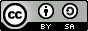### ANALISIS REPRESENTASI MATEMATIK SISWA SEKOLAH DASAR DALAM PENYELESAIAN MASALAH MATEMATIKA KONTEKSTUAL

#### Abstract

Abstract: The purpose of this study was to examine the forms of representation constructed by primary school students in solving mathematical problems. Representation is the basis or foundation of how a student could understand and use mathematical ideas. The forms of representation, such as charts, graphs, and symbols, are essentially a long process of learning mathematics, but unfortunately these representations are often thought of and studied in its final form. Actually, representations should be given as support in the process of understanding concepts, the associations of mathematics, mathematical communication, constructing arguments, and apply mathematical concepts in everyday life through modeling. This research showed that the forms of representation constructed by the students are extremely varied. They are constructed in tables, images, patterns, and in the formal forms of mathematics (the formula). This study was also revealed that some students are able to develop forms of representation using logical mathematical processes. Students begin to formulate a representation using known premise, set the table, make conjecture, and subsequently arrange a formal representation.

Keywords: mathematic representation, tables, charts, graphs, statements.

#### Full Text:

PDF

DOI: http://dx.doi.org/10.18269/jpmipa.v16i1.273

### Refbacks

• There are currently no refbacks.